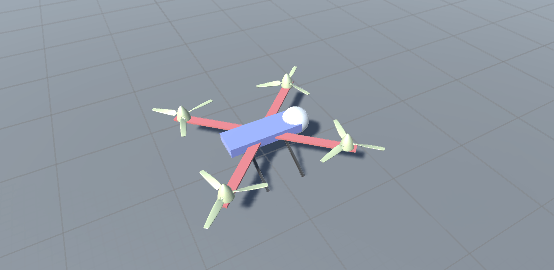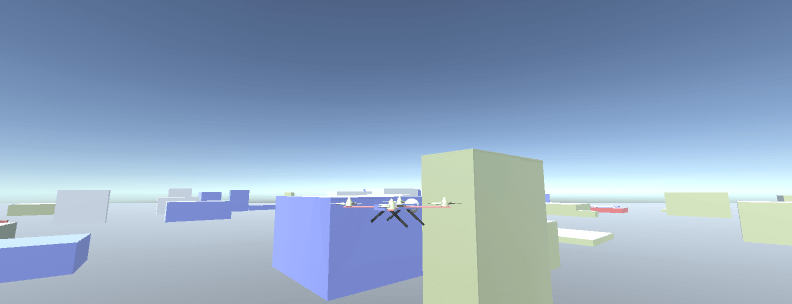# Minimize an error with a PID controller in Unity with C#

## 3. How to stabilize a quadcopter

This is a tutorial on how you can minimize an error with the help of a PID controller. This is a technique used in real-life quadcopters, which is like a helicopter but with four rotors.

The difference between the last tutorials and this one is that you will need more than one PID controller to stabilize the quadcopter. You will need at least two, but we will use three to be on the safe side. The three errors you will need to minimize are: roll angle, pitch angle, and yaw angle velocity. If you minimize these three errors, the quadcopter will be able to hover in the air. If you don't, the quadcopter will crash and burn.You need to build a simple quadcopter. Mine looks like this where the white sphere is there so we know which way is forward. I will refer to the different propellers as FL for front-left propeller, and so on.But we also will need make the quadcopter fly around and not just hover in the air. This is how a real quadcopter is doing it:

• Fly forward. Increase the propeller speed of the BL and BR propellers while decreasing the speed of the FL and FR propellers. To make it reverse, just do the opposite.
• Fly left. Increase the propeller speed of the FR and BR propellers while decreasing the speed of the FL and BL propellers. To make it fly right, just do the opposite.
• Rotate. Increase the propeller speed of the FR and BL propellers while decreasing the speed of the FL and BR propellers. To make it rotate in the other direction, just do the opposite. But to make this work in Unity, we will just add a torque to the helicopter because we don't have any real propellers so just adding a torque is much easier.

### Code

This is the main quadcopter script. To control the quadcopter we will use WASD and the arrow keys. A real quadcopter is using similar controls:There's a lot of different parameters you have to change to make everything work, and I've added mine in the comments in the code. My quadcopter's mass is 20 kg and its drag is 1, so add something like that to the rigidbody.

```using UnityEngine;
using System.Collections;

{
//The propellers
public GameObject propellerFR;
public GameObject propellerFL;
public GameObject propellerBL;
public GameObject propellerBR;

public float maxPropellerForce; //100
public float maxTorque; //1
public float throttle;
public float moveFactor; //5
//PID
public Vector3 PID_pitch_gains; //(2, 3, 2)
public Vector3 PID_roll_gains; //(2, 0.2, 0.5)
public Vector3 PID_yaw_gains; //(1, 0, 0)

//External parameters
public float windForce;
//0 -> 360
public float forceDir;

//The PID controllers
private PIDController PID_pitch;
private PIDController PID_roll;
private PIDController PID_yaw;

//Movement factors
float moveForwardBack;
float moveLeftRight;
float yawDir;

void Start()
{

PID_pitch = new PIDController();
PID_roll = new PIDController();
PID_yaw = new PIDController();
}

void FixedUpdate()
{

}

{
//Change throttle to move up or down
if (Input.GetKey(KeyCode.UpArrow))
{
throttle += 5f;
}
if (Input.GetKey(KeyCode.DownArrow))
{
throttle -= 5f;
}

throttle = Mathf.Clamp(throttle, 0f, 200f);

//Steering
//Move forward or reverse
moveForwardBack = 0f;

if (Input.GetKey(KeyCode.W))
{
moveForwardBack = 1f;
}
if (Input.GetKey(KeyCode.S))
{
moveForwardBack = -1f;
}

//Move left or right
moveLeftRight = 0f;

if (Input.GetKey(KeyCode.A))
{
moveLeftRight = -1f;
}
if (Input.GetKey(KeyCode.D))
{
moveLeftRight = 1f;
}

//Rotate around the axis
yawDir = 0f;

if (Input.GetKey(KeyCode.LeftArrow))
{
yawDir = -1f;
}
if (Input.GetKey(KeyCode.RightArrow))
{
yawDir = 1f;
}
}

{
//Calculate the errors so we can use a PID controller to stabilize
//Assume no error is if 0 degrees

//Pitch
//Returns positive if pitching forward
float pitchError = GetPitchError();

//Roll
//Returns positive if rolling left
float rollError = GetRollError() * -1f;

//Adapt the PID variables to the throttle
Vector3 PID_pitch_gains_adapted = throttle > 100f ? PID_pitch_gains * 2f : PID_pitch_gains;

//Get the output from the PID controllers
float PID_roll_output = PID_roll.GetFactorFromPIDController(PID_roll_gains, rollError);

//Calculate the propeller forces
//FR
float propellerForceFR = throttle + (PID_pitch_output + PID_roll_output);

propellerForceFR -= moveForwardBack * throttle * moveFactor;
propellerForceFR -= moveLeftRight * throttle;

//FL
float propellerForceFL = throttle + (PID_pitch_output - PID_roll_output);

propellerForceFL -= moveForwardBack * throttle * moveFactor;
propellerForceFL += moveLeftRight * throttle;

//BR
float propellerForceBR = throttle + (-PID_pitch_output + PID_roll_output);

propellerForceBR += moveForwardBack * throttle * moveFactor;
propellerForceBR -= moveLeftRight * throttle;

//BL
float propellerForceBL = throttle + (-PID_pitch_output - PID_roll_output);

propellerForceBL += moveForwardBack * throttle * moveFactor;
propellerForceBL += moveLeftRight * throttle;

//Clamp
propellerForceFR = Mathf.Clamp(propellerForceFR, 0f, maxPropellerForce);
propellerForceFL = Mathf.Clamp(propellerForceFL, 0f, maxPropellerForce);
propellerForceBR = Mathf.Clamp(propellerForceBR, 0f, maxPropellerForce);
propellerForceBL = Mathf.Clamp(propellerForceBL, 0f, maxPropellerForce);

//Add the force to the propellers

//Yaw
//Minimize the yaw error (which is already signed):

float PID_yaw_output = PID_yaw.GetFactorFromPIDController(PID_yaw_gains, yawError);

//First we need to add a force (if any)

//Then we need to minimize the error
}

{
Vector3 propellerUp = propellerObj.transform.up;

Vector3 propellerPos = propellerObj.transform.position;

//Debug
//Debug.DrawRay(propellerPos, propellerUp * 1f, Color.red);
}

//Pitch is rotation around x-axis
//Returns positive if pitching forward
private float GetPitchError()
{
float xAngle = transform.eulerAngles.x;

//Make sure the angle is between 0 and 360
xAngle = WrapAngle(xAngle);

//This angle going from 0 -> 360 when pitching forward
//So if angle is > 180 then it should move from 0 to 180 if pitching back
if (xAngle > 180f && xAngle < 360f)
{
xAngle = 360f - xAngle;

//-1 so we know if we are pitching back or forward
xAngle *= -1f;
}

return xAngle;
}

//Roll is rotation around z-axis
//Returns positive if rolling left
private float GetRollError()
{
float zAngle = transform.eulerAngles.z;

//Make sure the angle is between 0 and 360
zAngle = WrapAngle(zAngle);

//This angle going from 0-> 360 when rolling left
//So if angle is > 180 then it should move from 0 to 180 if rolling right
if (zAngle > 180f && zAngle < 360f)
{
zAngle = 360f - zAngle;

//-1 so we know if we are rolling left or right
zAngle *= -1f;
}

return zAngle;
}

//Wrap between 0 and 360 degrees
float WrapAngle(float inputAngle)
{
//The inner % 360 restricts everything to +/- 360
//+360 moves negative values to the positive range, and positive ones to > 360
//the final % 360 caps everything to 0...360
return ((inputAngle % 360f) + 360f) % 360f;
}

{
//Important to not use the quadcopters forward
Vector3 windDir = -Vector3.forward;

//Rotate it
windDir = Quaternion.Euler(0, forceDir, 0) * windDir;

//Debug
//Is showing in which direction the wind is coming from
//center of quadcopter is where it ends and is blowing in the direction of the line
Debug.DrawRay(transform.position, -windDir * 3f, Color.red);
}
}
```

Next up is the PID controller. You can change a lot of settings in a PID controller. For example, when calculating the derivative of the error you can sometimes use an error from two time steps back and sometimes you use an error from the last time step. When calculating the sum of the errors, you can sometimes use a maximum value to not make the sum grow too big.

```using UnityEngine;
using System.Collections;

public class PIDController
{
float error_old = 0f;
//The controller will be more robust if you are using a further back sample
float error_old_2 = 0f;
float error_sum = 0f;
//If we want to average an error as input
float error_sum2 = 0f;

//PID parameters
public float gain_P = 0f;
public float gain_I = 0f;
public float gain_D = 0f;
//Sometimes you have to limit the total sum of all errors used in the I
private float error_sumMax = 20f;

public float GetFactorFromPIDController(float error)
{
float output = CalculatePIDOutput(error);

return output;
}

//Use this when experimenting with PID parameters
public float GetFactorFromPIDController(float gain_P, float gain_I, float gain_D, float error)
{
this.gain_P = gain_P;
this.gain_I = gain_I;
this.gain_D = gain_D;

float output = CalculatePIDOutput(error);

return output;
}

//Use this when experimenting with PID parameters and the gains are stored in a Vector3
public float GetFactorFromPIDController(Vector3 gains, float error)
{
this.gain_P = gains.x;
this.gain_I = gains.y;
this.gain_D = gains.z;

float output = CalculatePIDOutput(error);

return output;
}

private float CalculatePIDOutput(float error)
{
//The output from PID
float output = 0f;

//P
output += gain_P * error;

//I
error_sum += Time.fixedDeltaTime * error;

//Clamp the sum
this.error_sum = Mathf.Clamp(error_sum, -error_sumMax, error_sumMax);

//Sometimes better to just sum the last errors
//float averageAmount = 20f;

//CTE_sum = CTE_sum + ((CTE - CTE_sum) / averageAmount);

output += gain_I * error_sum;

//D
float d_dt_error = (error - error_old) / Time.fixedDeltaTime;

//Save the last errors
this.error_old_2 = error_old;

this.error_old = error;

output += gain_D * d_dt_error;

return output;
}
}

```

And that's it! You can now take sneaky pictures of your neighbors: